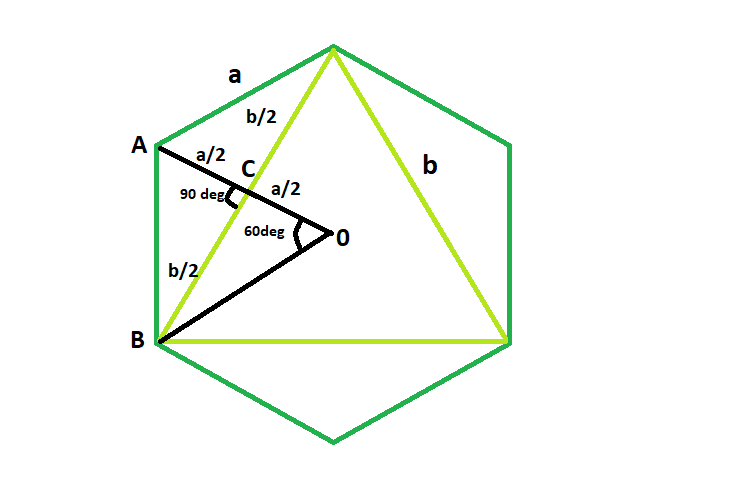# Area of the Largest Triangle inscribed in a Hexagon

Given here is a regular hexagon, of side length a, the task is to find the area of the biggest triangle that can be inscribed within it.

Examples:

```Input:  a = 6
Output: area = 46.7654

Input: a = 8
Output: area = 83.1384
```## Recommended: Please try your approach on {IDE} first, before moving on to the solution.

Approach:

It is very clear that the biggest triangle that can be inscribed within the hexagon is an equilateral triangle.
In triangle ACD,
following pythagorus theorem,
(a/2)^2 + (b/2)^2 = a^2
b^2/4 = 3a^2/4
So, b = a√3

Therefore, area of the triangle, A = √3(a√3)^2/4= 3√3a^2/4

Below is the implementation of the above approach:

## C++

 `// C++ Program to find the biggest triangle ` `// which can be inscribed within the hexagon ` `#include ` `using` `namespace` `std; ` ` `  `// Function to find the area ` `// of the triangle ` `float` `trianglearea(``float` `a) ` `{ ` ` `  `    ``// side cannot be negative ` `    ``if` `(a < 0) ` `        ``return` `-1; ` ` `  `    ``// area of the triangle ` `    ``float` `area = (3 * ``sqrt``(3) * ``pow``(a, 2)) / 4; ` ` `  `    ``return` `area; ` `} ` ` `  `// Driver code ` `int` `main() ` `{ ` `    ``float` `a = 6; ` `    ``cout << trianglearea(a) << endl; ` ` `  `    ``return` `0; ` `} `

## Java

 `// Java Program to find the biggest triangle ` `// which can be inscribed within the hexagon ` ` `  `import` `java.io.*; ` ` `  `class` `GFG { ` `     `  `// Function to find the area ` `// of the triangle ` `static` `double` `trianglearea(``double` `a) ` `{ ` ` `  `    ``// side cannot be negative ` `    ``if` `(a < ``0``) ` `        ``return` `-``1``; ` ` `  `    ``// area of the triangle ` `    ``double` `area = (``3` `* Math.sqrt(``3``) * Math.pow(a, ``2``)) / ``4``; ` ` `  `    ``return` `area; ` `} ` ` `  `    ``public` `static` `void` `main (String[] args) { ` `        ``double` `a = ``6``; ` `        ``System.out.println (trianglearea(a)); ` ` `  `    ``} ` `//This Code is contributed by Sachin.. ` `     `  `} `

## Python3

 `# Python3 Program to find the biggest triangle ` `# which can be inscribed within the hexagon ` `import` `math ` ` `  `# Function to find the area ` `# of the triangle ` `def` `trianglearea(a): ` ` `  `    ``# side cannot be negative ` `    ``if` `(a < ``0``): ` `        ``return` `-``1``; ` ` `  `    ``# area of the triangle ` `    ``area ``=` `(``3` `*` `math.sqrt(``3``) ``*` `math.``pow``(a, ``2``)) ``/` `4``; ` ` `  `    ``return` `area; ` ` `  `# Driver code ` `a ``=` `6``; ` `print``(trianglearea(a)) ` ` `  `# This code is contributed  ` `# by Akanksha Rai `

## C#

 `// C# Program to find the biggest triangle  ` `// which can be inscribed within the hexagon ` ` `  `using` `System; ` ` `  `class` `GFG {  ` `     `  `// Function to find the area  ` `// of the triangle  ` `static` `double` `trianglearea(``double` `a)  ` `{  ` ` `  `    ``// side cannot be negative  ` `    ``if` `(a < 0)  ` `        ``return` `-1;  ` ` `  `    ``// area of the triangle  ` `    ``double` `area = (3 * Math.Sqrt(3) * Math.Pow(a, 2)) / 4;  ` ` `  `    ``return` `Math.Round(area,4);  ` `}  ` ` `  `    ``public` `static` `void` `Main () {  ` `        ``double` `a = 6;  ` `        ``Console.WriteLine(trianglearea(a));  ` ` `  `    ``}  ` `        ``// This code is contributed by Ryuga ` ` `  `}  `

## PHP

 ` `

Output:

```46.7654
```

Attention reader! Don’t stop learning now. Get hold of all the important DSA concepts with the DSA Self Paced Course at a student-friendly price and become industry ready.

My Personal Notes arrow_drop_upCheck out this Author's contributed articles.

If you like GeeksforGeeks and would like to contribute, you can also write an article using contribute.geeksforgeeks.org or mail your article to contribute@geeksforgeeks.org. See your article appearing on the GeeksforGeeks main page and help other Geeks.

Please Improve this article if you find anything incorrect by clicking on the "Improve Article" button below.